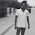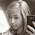## Saturday, March 12, 2011

### VBScript: Listing the prime numbers

```'VBScript program to display the prime numbers
PrimeNumberLimit = inputbox("Enter the range till (ex: 50):")
'Getting the limit as an input from the user

'Initialising the list of prime numbers

PrimeFlag=True
' Initialising a Flag variable

If  Ctr1>=4 Then

For Ctr2=2 to Ctr1/2

If Ctr1 mod Ctr2 = 0 Then 'Checking the reminder

PrimeFlag=False
'Not a prime number

End If

Next

End If

If PrimeFlag=True Then

'Collecting the prime numbers

End If

Next

'Displaying the result

```

Program Explained

Line 2: Getting the input from the user and storing it in a variable.

Sample output:

Line 5: Initializing the list of prime numbers in a string.

Line 8: Checking for all the numbers starting from 1 till user's input

Line 10: Initializing a flag variable.

Line 13: Excluding 1,2,3 the first three prime numbers.

Lines 15-22: Checking for the reminder, and if it is zero we conclude it is not a prime number.

Lines 28-33: Collecting all the prime numbers in a string.

Line 37: Displaying the list of prime numbers.

Result:

More such VB Scripts here.

1.'Getting the limit as an input from the user
PrimeNumberLimit = Inputbox("Enter the range to check Prime no : ")

'Initialising the list of prime numbers

For Ctr1=1 to PrimeNumberLimit step 2 'check only odd nos
PrimeFlag=True ' Initialising a Flag variable
If Ctr1>=4 Then
For Ctr2=2 to Ctr1/2
If Ctr1 mod Ctr2 = 0 Then 'Checking the reminder
PrimeFlag=False 'Not a prime number
Exit For 'no need to check further
End If
Next
End If
If PrimeFlag=True Then
End If

Next

'Displaying the result

email: rajinder500@gmail.com

1.is 2 a primenumber or not?

2.@ Anonymous : yes it is, in fact 2 is the only even prime number.

2.Hi , above code shows 1 as Prime number But Wiki and other Google search page indicates 1 is Not a Prime Number ...
Wiki : A prime number (or a prime) is a natural number greater than 1 that has no positive divisors other than 1 and itself.

Se we have to start count of "Ctr1" variable from 2 i think so ....

3.for j=1 to 50 step 1
c=0
for i=1 to j step 1
if j mod i= 0 then 'divisibility ruke for prime remeinder is 0 only for 2 values'
c=c+1
end if
next
if c= 2 then
temp=temp&j&vbnewline
end if
next
msgbox temp

4.for j=1 to 50 step 1
c=0
for i=1 to j step 1
if j mod i= 0 then 'using divisiblity rule of prime no. which have deir remainder 0 only for 2 values d no. n itself
c=c+1
end if
next
if c= 2 then
temp=temp&j&vbnewline
end if
next
msgbox temp

5.for j=1 to 50 step 1
c=0
for i=1 to j step 1
if j mod i= 0 then
c=c+1
end if
next
if c= 2 then
temp=temp&j&vbnewline
end if
next
msgbox temp

6.' Performance improvements to generate primes significantly faster than the previous script
'VBScript program to display the prime numbers
PrimeNumberLimit = inputbox("Enter the range till (ex: 50):")
'Getting the limit as an input from the user
wscript.echo Now()
' echo the start time of the script
'Initialising the list of prime numbers

' Initialise list with numbers we now to be prime but do not want the loop testing.

PrimeFlag=True
' Initialising the Prime Flag variable

If Right(isPrime,1) = "1" or Right(isPrime,1) = "3" or Right(isPrime,1) = "7" or Right(isPrime,1) = "9" Then
' We know that prime numbers beyond 5 have a last digit which is either 1, 3, 7, or 9
' as all numbers greater than 5 whose last digit is 0, 2, 4, 6, 8 are divisible by 2 and all
' numbers greater than 5 whose last digit is 5 is divisible by 5.

For primeDivisor=2 to round(sqr(isPrime))+1
' We don't need to use all the numbers to 1/2 of the number to be tested - we only need
' test to the whole number nearest its square root.

If isPrime mod primeDivisor = 0 Then 'Checking the reminder

PrimeFlag=False
'Not a prime number
Exit For
End If

Next

Else
PrimeFlag=False
' If the number's last digit is 0, 2, 4, 5, 6, or 8 then it is not prime.
End If

If PrimeFlag=True Then
i = i + 1
'wscript.echo isPrime
'Collecting the prime numbers
if i mod 20 = 0 then